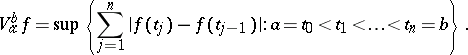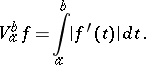# Total variation of a function

(diff) ← Older revision | Latest revision (diff) | Newer revision → (diff)
Jump to: navigation, search

The same as the variation of a function of one variable. The total variation of a real-valued function is the sum of its positive variation (cf. Positive variation of a function) and negative variation (cf. Negative variation of a function).

#### Comments

Ifis a complex-valued function on, then its total variation overis the numberIfis also continuous, then this number is the same as the length of the arc in the complex plane that is parametrized by. Ifis absolutely continuous on, thenHow to Cite This Entry:
Total variation of a function. Encyclopedia of Mathematics. URL: http://encyclopediaofmath.org/index.php?title=Total_variation_of_a_function&oldid=28014
This article was adapted from an original article by B.I. Golubov (originator), which appeared in Encyclopedia of Mathematics - ISBN 1402006098. See original article